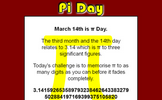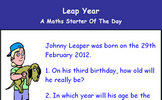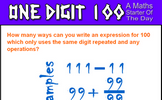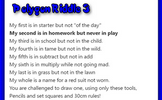# Working with circles

### Term 2 starting in week 3 :: Estimated time: 2 weeks

• Recognise and label parts of a circle (review)
• Calculate fractional parts of a circle
• Calculate the length of an arc
• Calculate the area of a sector
• Understand and use the volume of a cylinder and cone
• Understand and use the volume of a sphere
• Understand and use the surface area of a sphere
• Understand and use the surface area of a cylinder and cone

For higher-attaining pupils:

• Circle theorem: Angles at the centre and circumference
• Circle theorem: Angles in a semicircle
• Circle theorem: Angles in the same segment
• Circle theorem: Angles in a cyclic quadrilateral
• Solve area and volume problems involving similar shapes

This page should remember your ticks from one visit to the next for a period of time. It does this by using Local Storage so the information is saved only on the computer you are working on right now.

## Lesson Starters

Here are some suggestions for whole-class, projectable resources which can be used at the beginnings of each lesson in this block.

### 1st Lesson#### Pi Day

March 14th is Pi Day. The third month and the 14th day relates to 3.14 which is pi to three significant figures. How many figures of pi can you memorise?

### 2nd Lesson#### Leap Year

A question about the birthdays of a child born on the 29th February.

### 3rd Lesson#### On The Double

Double the numbers given in the table.

### 4th Lesson#### One Digit 100

How many ways can you write an expression for 100 which only uses the same digit repeated and any operations?

### 5th Lesson#### Plus

A number puzzle suitable for children with a wide range of abilities.

### 6th Lesson#### Polygon Riddle 3

A 'My first is in...' riddle that describes a geometrical shape. Can you construct it?

Some of the Starters above are to reinforce concepts learnt, others are to introduce new ideas while others are on unrelated topics designed for retrieval practice or and opportunity to develop problem-solving skills.

White Rose ResourcesEnd of block assessments provide a quick progress check at the end of each block of learning to make sure students have understood the content covered. This Scheme of Learning was produced by White Rose Maths and is used here with permission granted on 30th June 2021.For All: